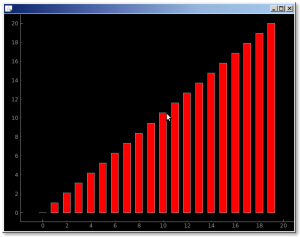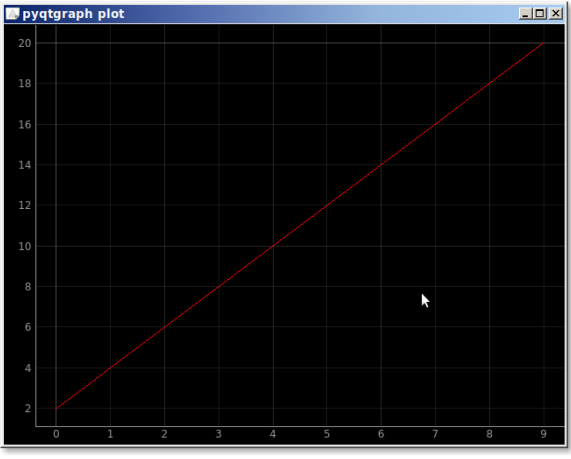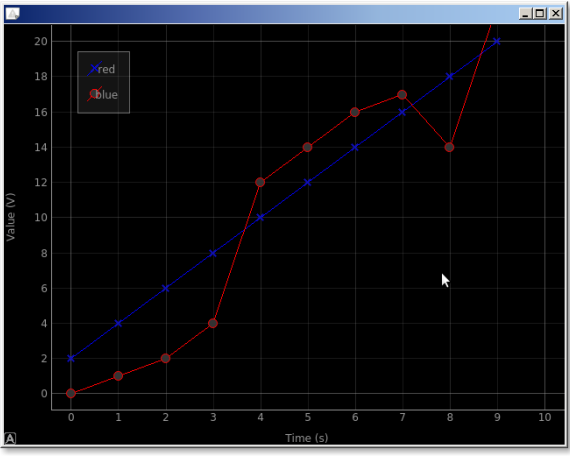tag: pyqtgraph | Python Tutorial

# Tag: pyqtgraph

## pyqtgraph bar chart

The module pyqtgraph supports all kinds of charts and scientific graphics. Underneath, pyqtgraph uses PyQt4 / PySide and numpy. Its been tested to work on Linux, Windows, and OSX.

In this article we’ll create an example bar chart. The output will be this awesome chart:Related course:

## pyqtgraph barchart

We create the data to plot (x y1) first. y1 is a list of 20 floats that we create using the numpy method linspace.

Then create the barchart using the method BarGraphItem(), where the parameters are x, y, bar width and color.

## pyqtgraph plot

A simple plot can be created with the module pyqtgraph. Mind you, it’s one of the libraries for plotting, there are others like matplotlib.

We start with importing pyqtgraph and defing the plotting data (x and y). Then we plot the data using pg.plot().Related course:

## pyqtgraph plot

The example below creates a plot using pyqtgraph.

We can set additional properties of the chart like the labels, add a legend, the colors.
The output will look like this:In code its like this:

1